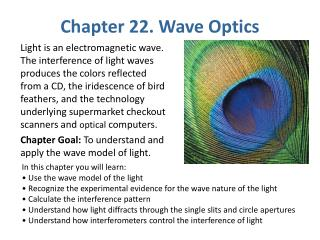# Chapter 22. Wave Optics - PowerPoint PPT PresentationDownload PresentationChapter 22. Wave Optics

Chapter 22. Wave OpticsDownload Presentation## Chapter 22. Wave Optics

- - - - - - - - - - - - - - - - - - - - - - - - - - - E N D - - - - - - - - - - - - - - - - - - - - - - - - - - -
##### Presentation Transcript

1. Chapter 22. Wave Optics Light is an electromagnetic wave. The interference of light waves produces the colors reflected from a CD, the iridescence of bird feathers, and the technology underlying supermarket checkout scanners and optical computers. Chapter Goal: To understand and apply the wave model of light. In this chapter you will learn: • Use the wave model of the light • Recognize the experimental evidence for the wave nature of the light • Calculate the interference pattern • Understand how light diffracts through the single slits and circle apertures • Understand how interferometers control the interference of light

2. Chapter 22. Wave Optics Topics: • Light and Optics • The Interference of Light • The Diffraction Grating • Single-Slit Diffraction • Circular-Aperture Diffraction • Interferometers

3. Stop to think 22.1 p673Stop to think 22.2 p676Stop to think 22.3 p680Stop to think 22.4 p684Stop to think 22.5 p688 Example 22.2 p676 Example 22.3 p680 Example 22.4 p683 Example 22.6 p685

5. Models of Light • The wave model: under many circumstances, light exhibits the same behavior as sound or water waves. The study of light as a wave is called wave optics. • The ray model: The properties of prisms, mirrors, and lenses are best understood in terms of light rays. The ray model is the basis of ray optics. • The photon model: In the quantum world, light behaves like neither a wave nor a particle. Instead, light consists of photons that have both wave-like and particle-like properties. This is the quantum theory of light.

6. Conditions for Interference The sources must be: 􀂆 A) coherent; 􀂆 B) monochromatic

7. Δr = dsinθ = mλ m = 0, 1, 2, 3 …… bright fringe Δr = dsinθ = (m+1/2) λ m = 0, 1, 2, 3 …… dark fringe

8. The intensity of the double-slit I (double) = 4I1, here I1 is the light intensity of a single slit

9. Diffraction • Diffraction: • Light spreads beyond the narrow path defined by the slit into regions that would be in shadow if light traveled in straight lines Diffraction Pattern Diffraction Geometric Optics - if Diffraction and Interference are closely related; Diffraction Patterns are due to Interference

10. Single-Slit Diffraction

11. Angle θ locates the first minimum in the intensity, A math. Analysis of circular diffraction Find θ = 1.22 λ/D, where D is the diameter of the circular opening.

12. Circular-Aperture Diffraction Light of wavelength λ passes through a circular aperture of diameter D, and is then incident on a viewing screen a distance L behind the aperture, L>>D. The diffraction pattern has a circular central maximum, surrounded by a series of secondary bright fringes shaped like rings. The angle of the first minimum in the intensity is The width of the central maximum on the screen is

14. The Diffraction Grating

15. The Diffraction Grating Suppose we were to replace the double slit with an opaque screen that has N closely spaced slits. When illuminated from one side, each of these slits becomes the source of a light wave that diffracts, or spreads out, behind the slit. Such a multi-slit device is called a diffraction grating. Bright fringes will occur at angles θm, such that λ/d is not small, so we do not use small angle approximation The y-positions of these fringes will occur at The intensities of the bright fringes I(max) = N2 I1

16. The Michelson Interferometer The path-length difference Between two wave Δr =2L2 - 2L1

17. Measuring Indices of Refraction A Michelson interferometer can be used to measure indices of refraction of gases. A cell of thickness d is inserted into one arm of the cell. When the cell contains a vacuum, the number of wavelengths inside the cell is When the cell is filled with a specific gas, the number of wavelengths spanning the distance d is Filling the cell has increased the lower path by wavelengths. By counting fringe shifts as the cell is filled, one can determine n.

18. General Principles

19. Applications

20. Applications

21. Suppose the viewing screen in the figure is moved closer to the double slit. What happens to the interference fringes? • They fade out and disappear. • They get out of focus. • They get brighter and closer together. • They get brighter and farther apart. • They get brighter but otherwise do not change. C

22. White light passes through a diffraction grating and forms rainbow patterns on a screen behind the grating. For each rainbow, • the red side is farthest from the center of the screen, the violet side is closest to the center. • the red side is closest to the center of the screen, the violet side is farthest from the center. • the red side is on the left, the violet side on the right. • the red side is on the right, the violet side on the left. A